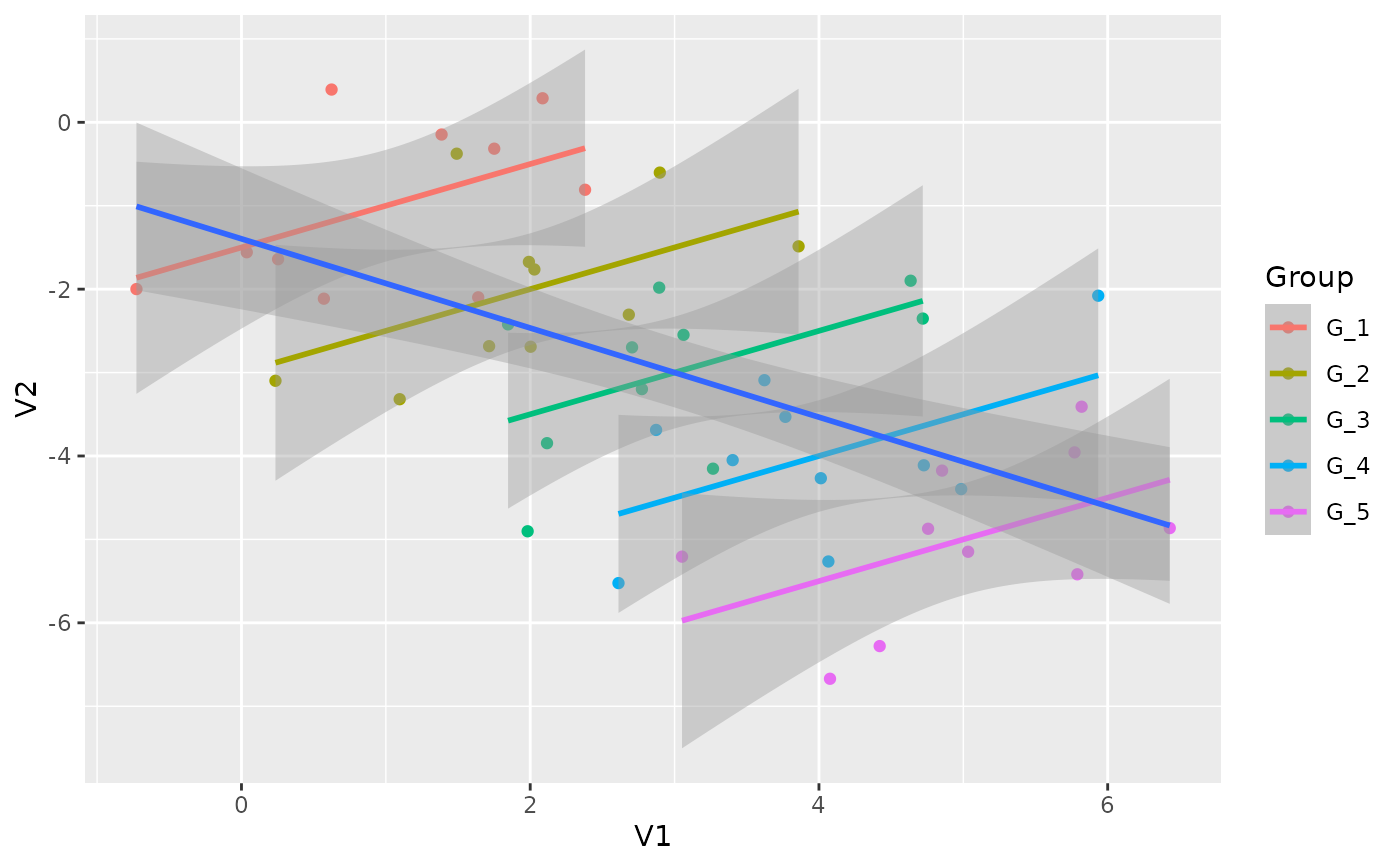Simpson's paradox, or the Yule-Simpson effect, is a phenomenon in probability and statistics, in which a trend appears in several different groups of data but disappears or reverses when these groups are combined.

## Usage

simulate_simpson(
n = 100,
r = 0.5,
groups = 3,
difference = 1,
group_prefix = "G_"
)

## Arguments

n

The number of observations for each group to be generated (minimum 4).

r

A value or vector corresponding to the desired correlation coefficients.

groups

Number of groups (groups can be participants, clusters, anything).

difference

Difference between groups.

group_prefix

The prefix of the group name (e.g., "G_1", "G_2", "G_3", ...).

A dataset.

## Examples

data <- simulate_simpson(n = 10, groups = 5, r = 0.5)

if (require("ggplot2")) {
ggplot(data, aes(x = V1, y = V2)) +
geom_point(aes(color = Group)) +
geom_smooth(aes(color = Group), method = "lm") +
geom_smooth(method = "lm")
}
#> geom_smooth() using formula = 'y ~ x'
#> geom_smooth() using formula = 'y ~ x'